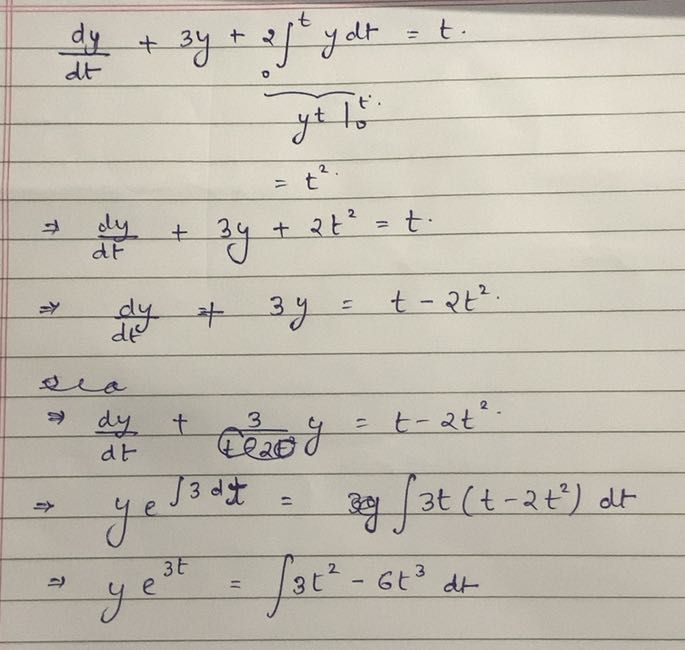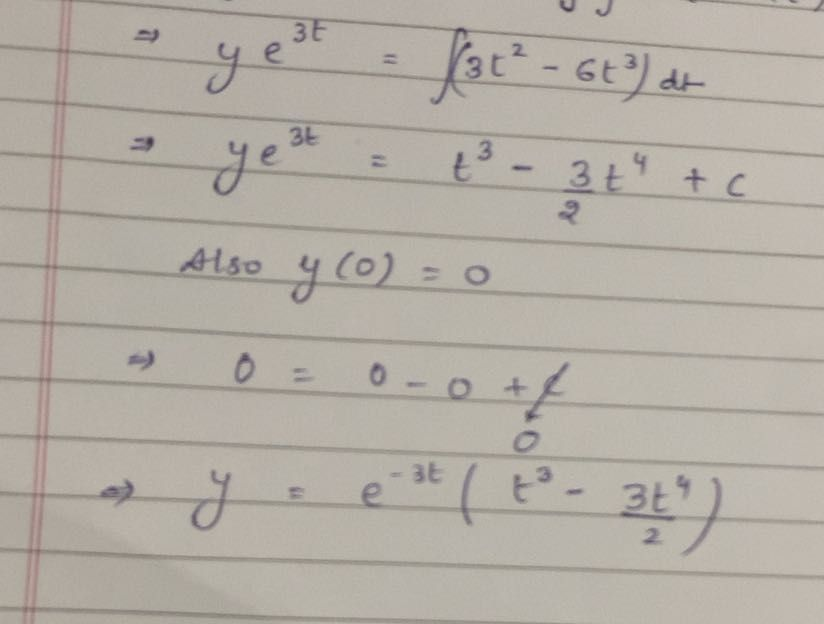Symbol
ProblemUsing Laplace transform solve, $\dfrac {dy} {d1}+3y+2\int _{0}ydt=1,y\left(0\right)=0$
Calculus
Search count: 105
SolutionQanda teacher - RishabhStudent
thank you sir